### 一、题目描述

输入：
[2, 3, 1, 0, 2, 5, 3]

2 <= n <= 100000

### 四、图片描述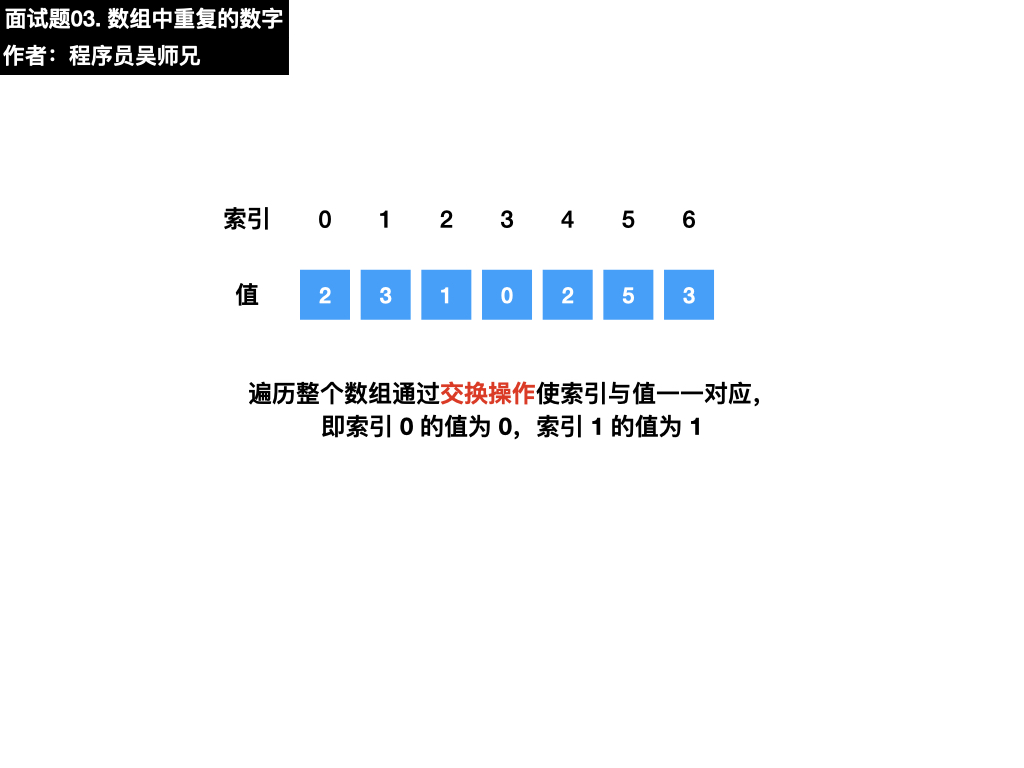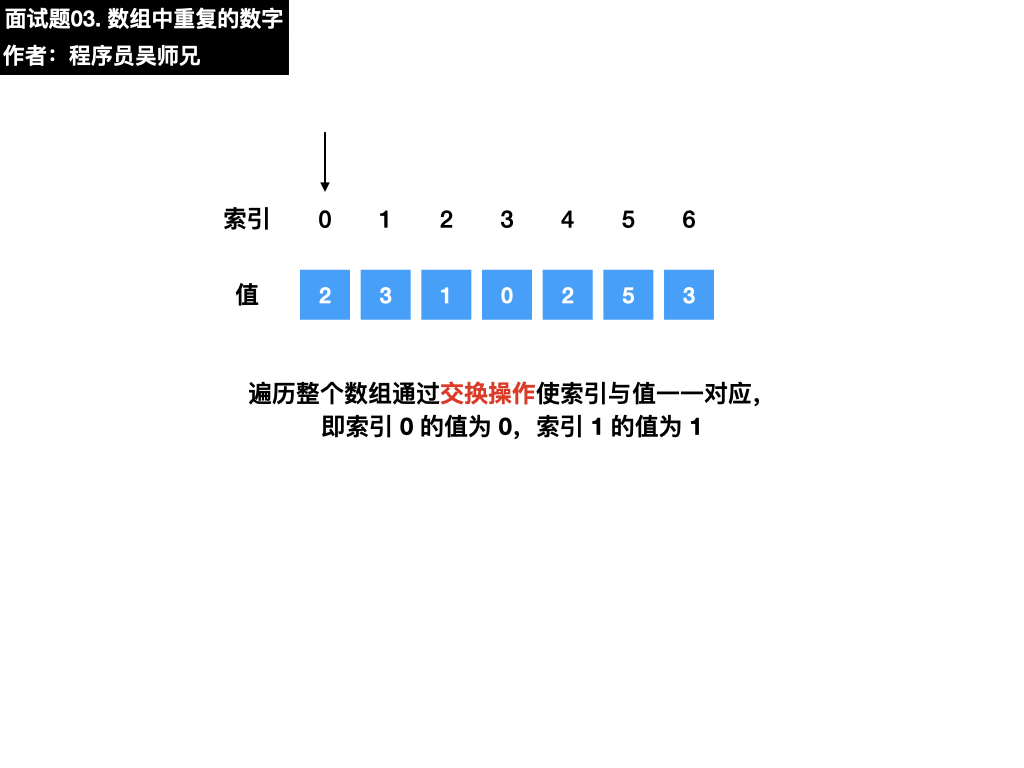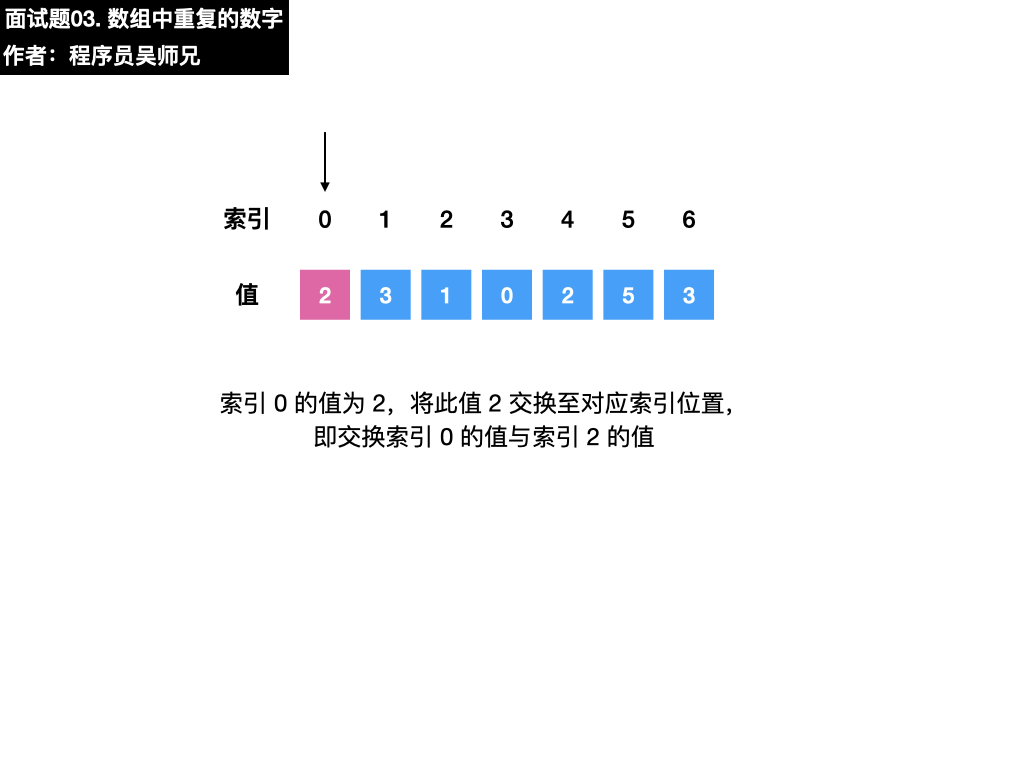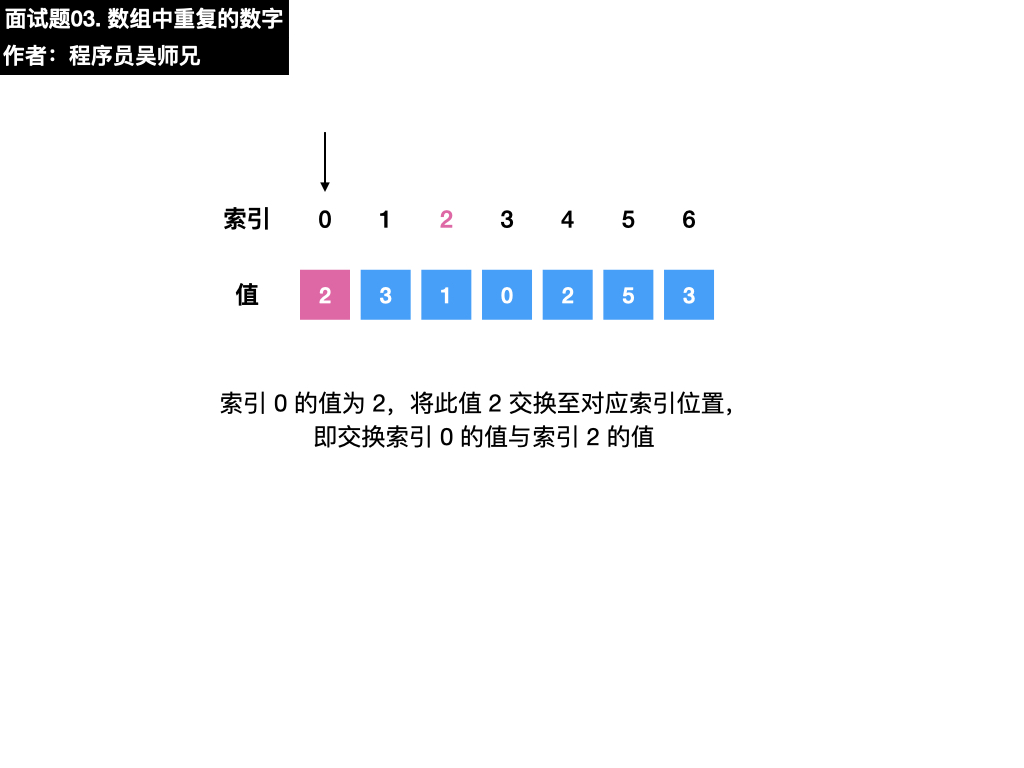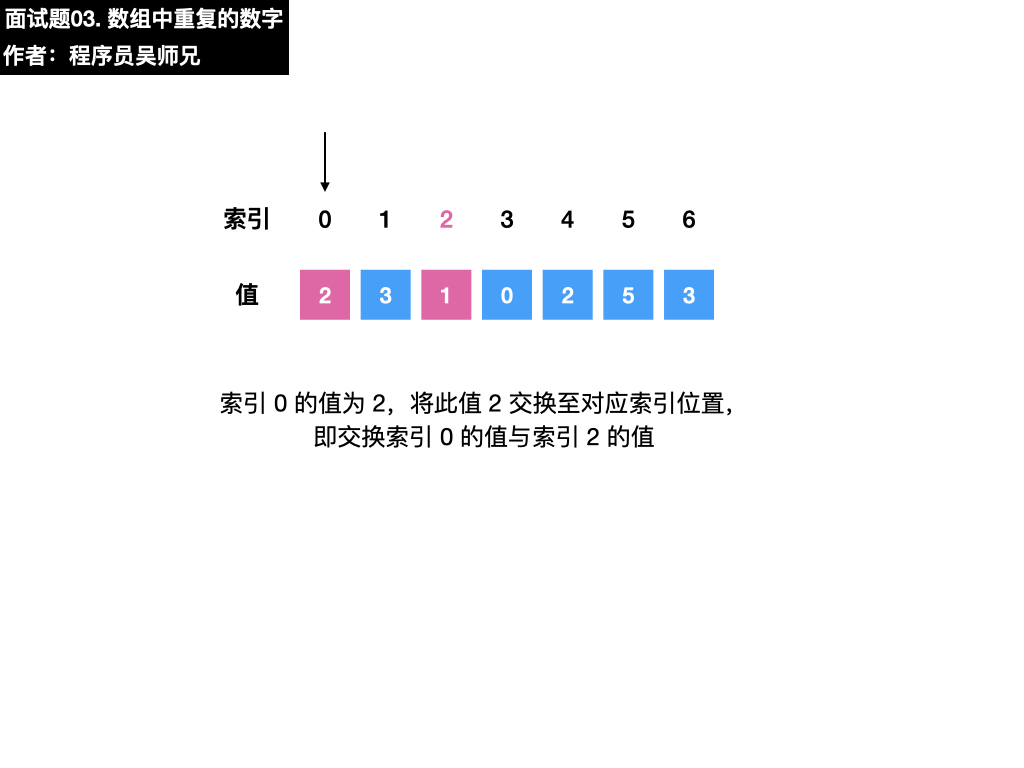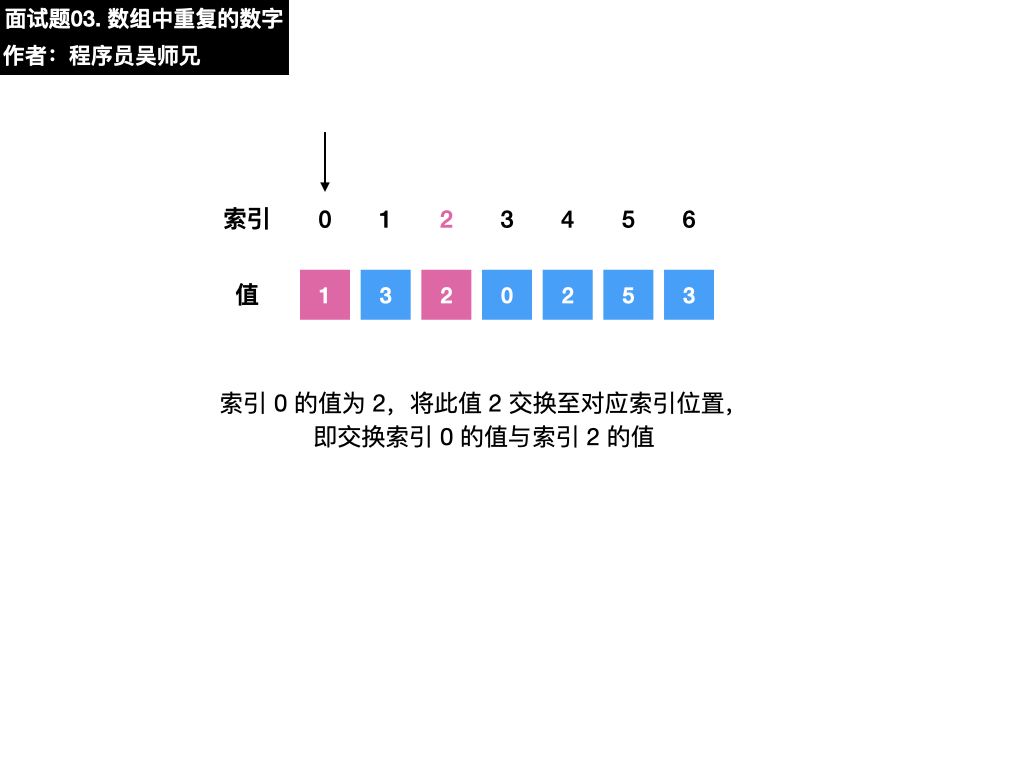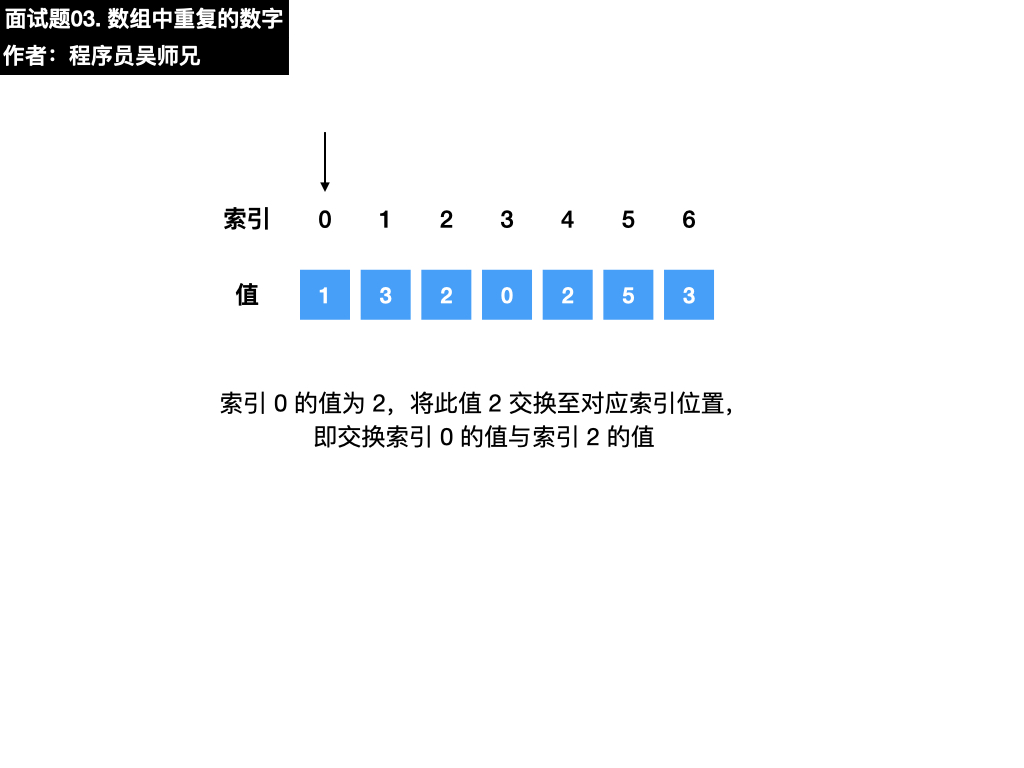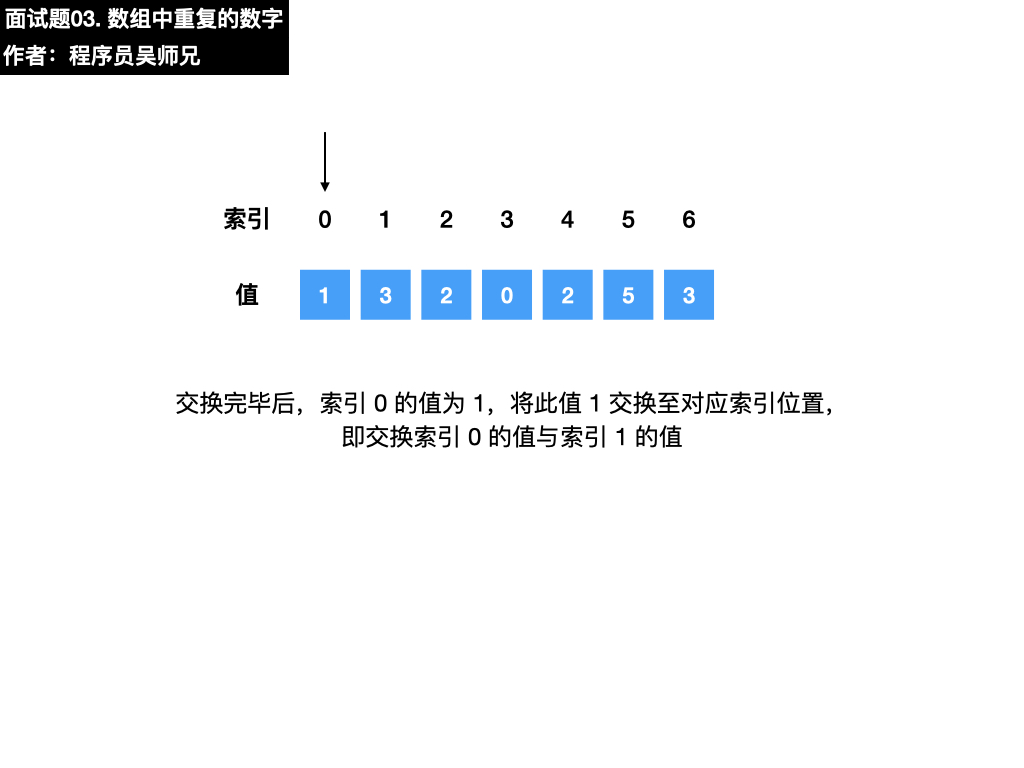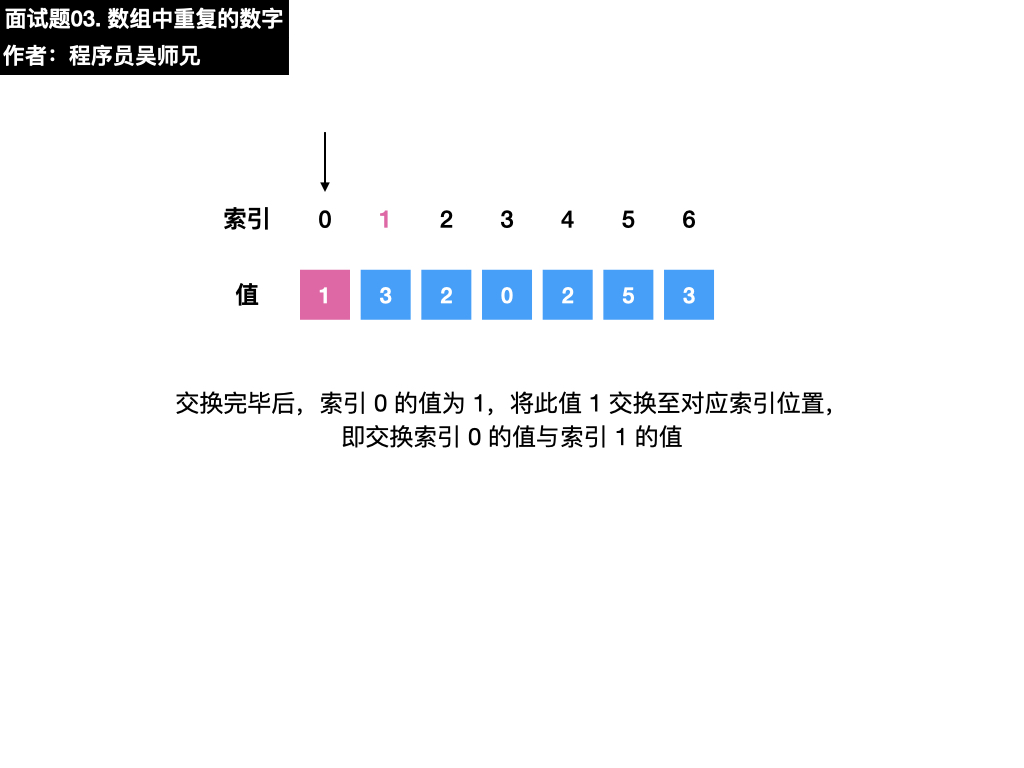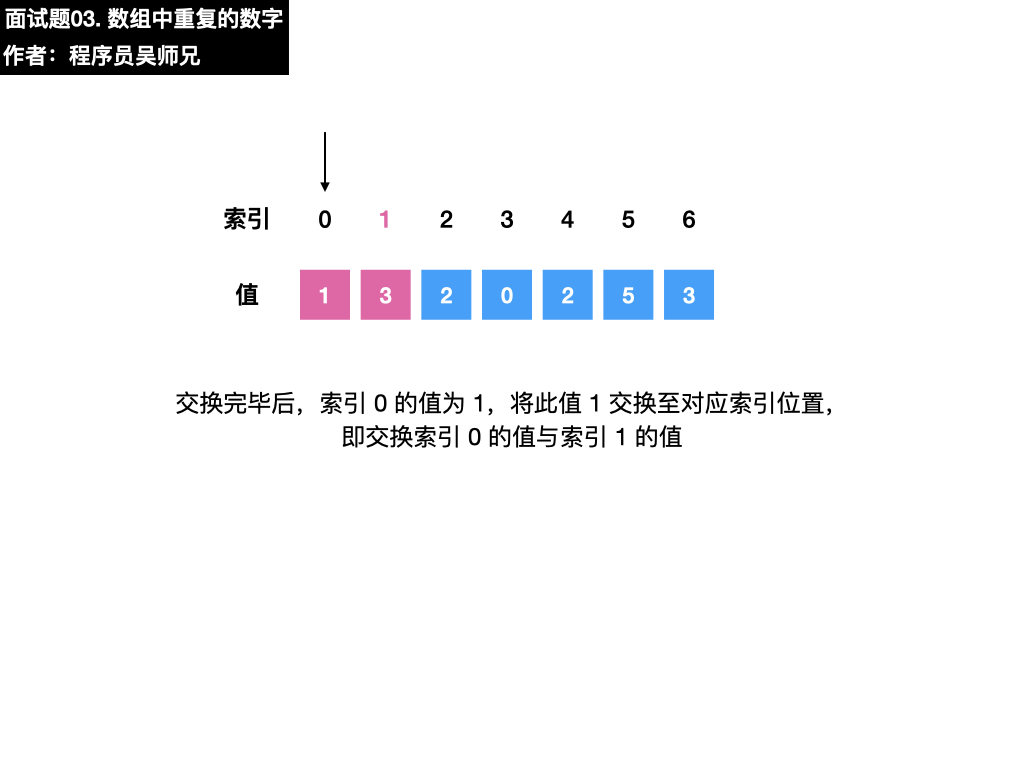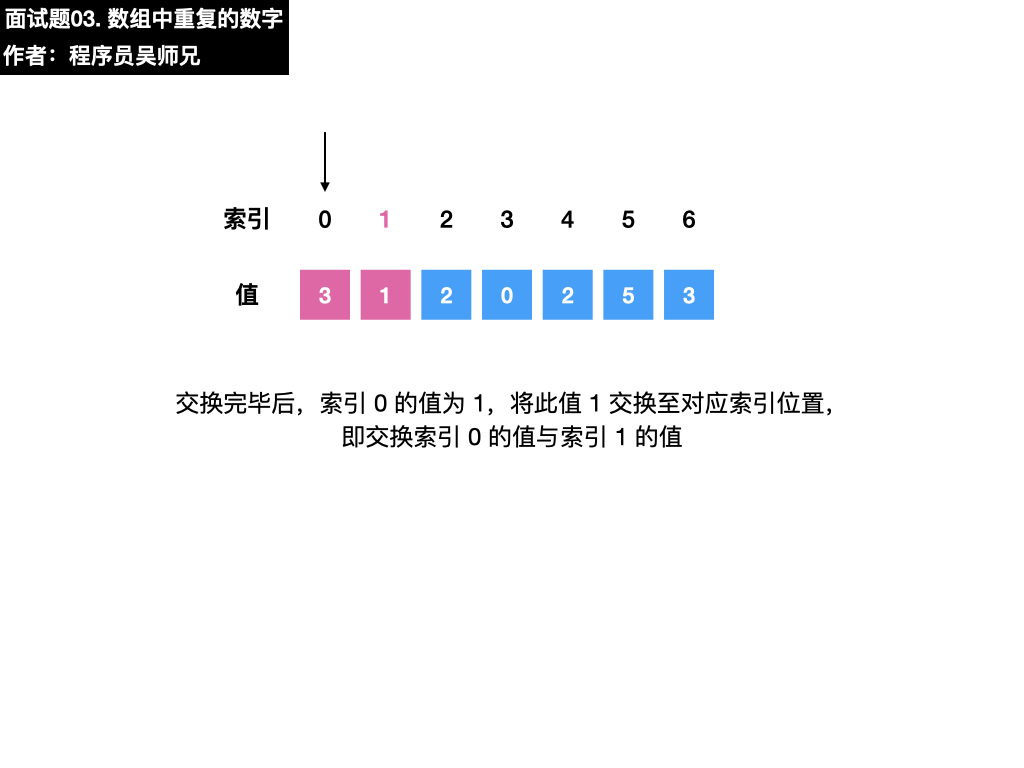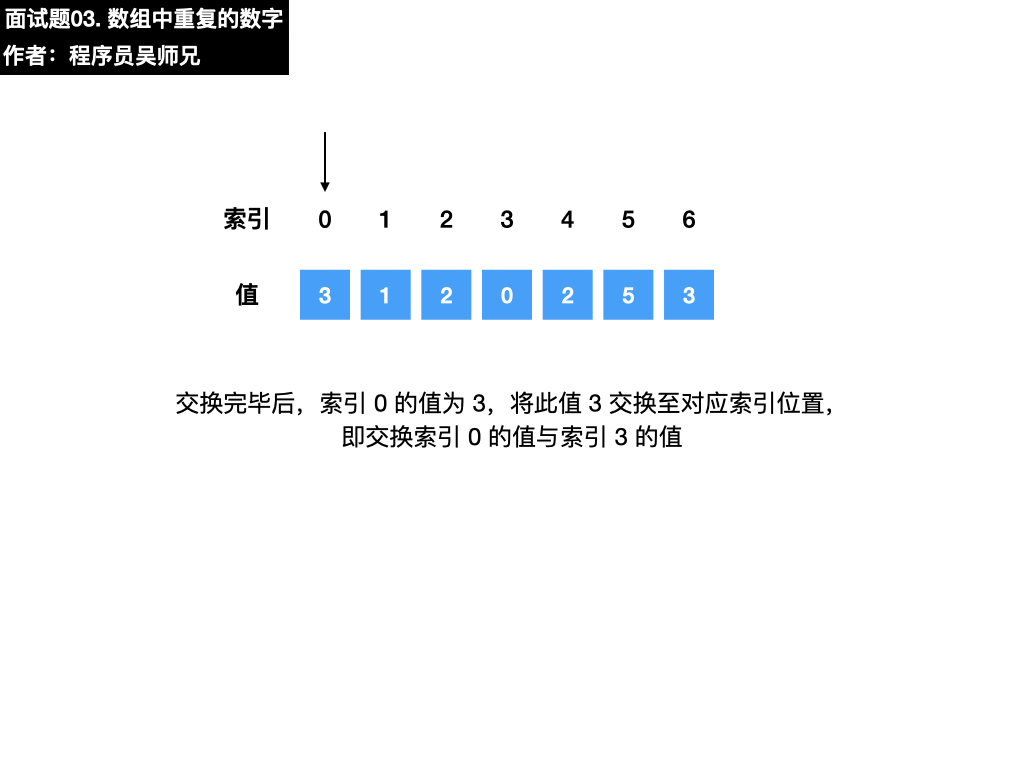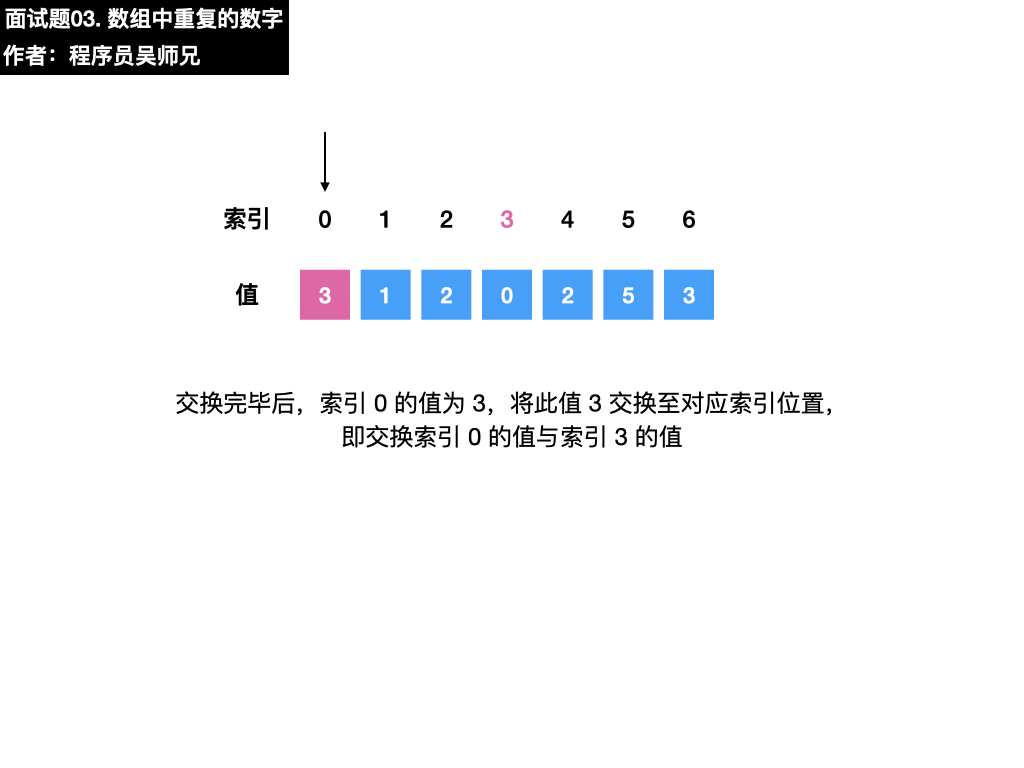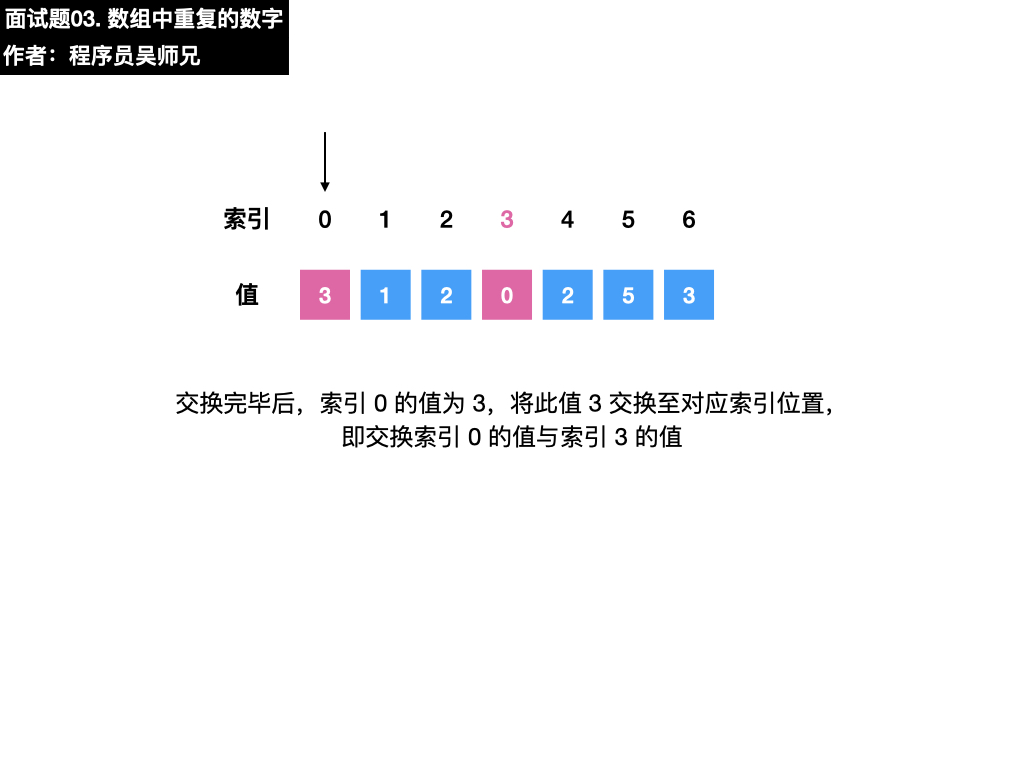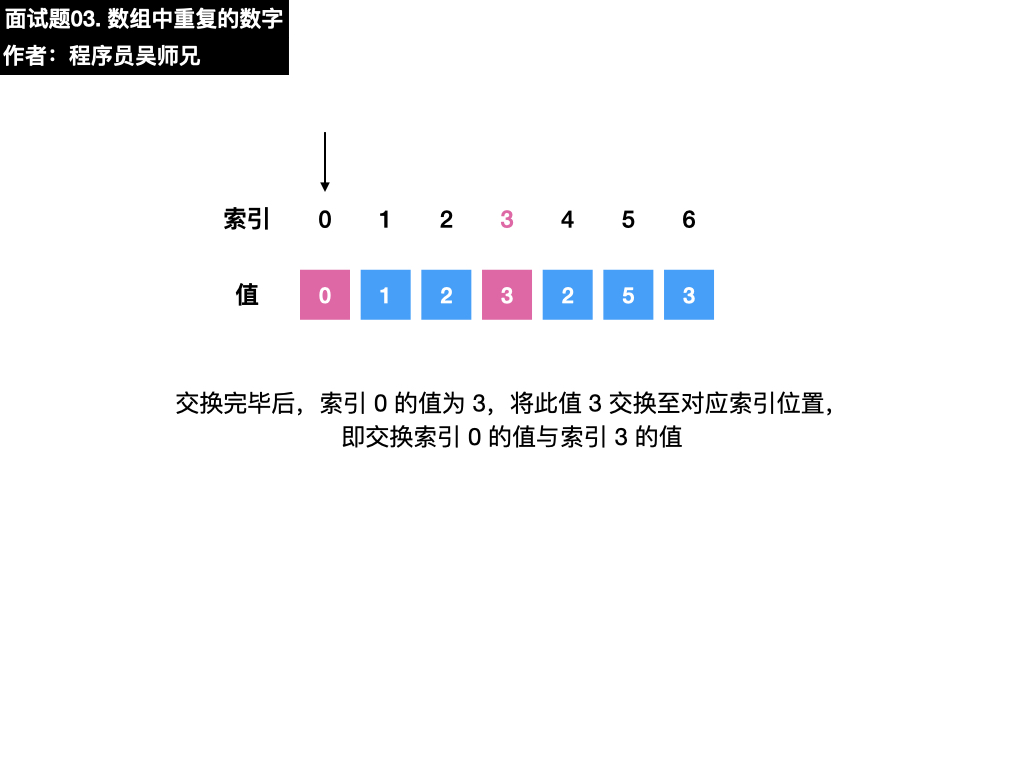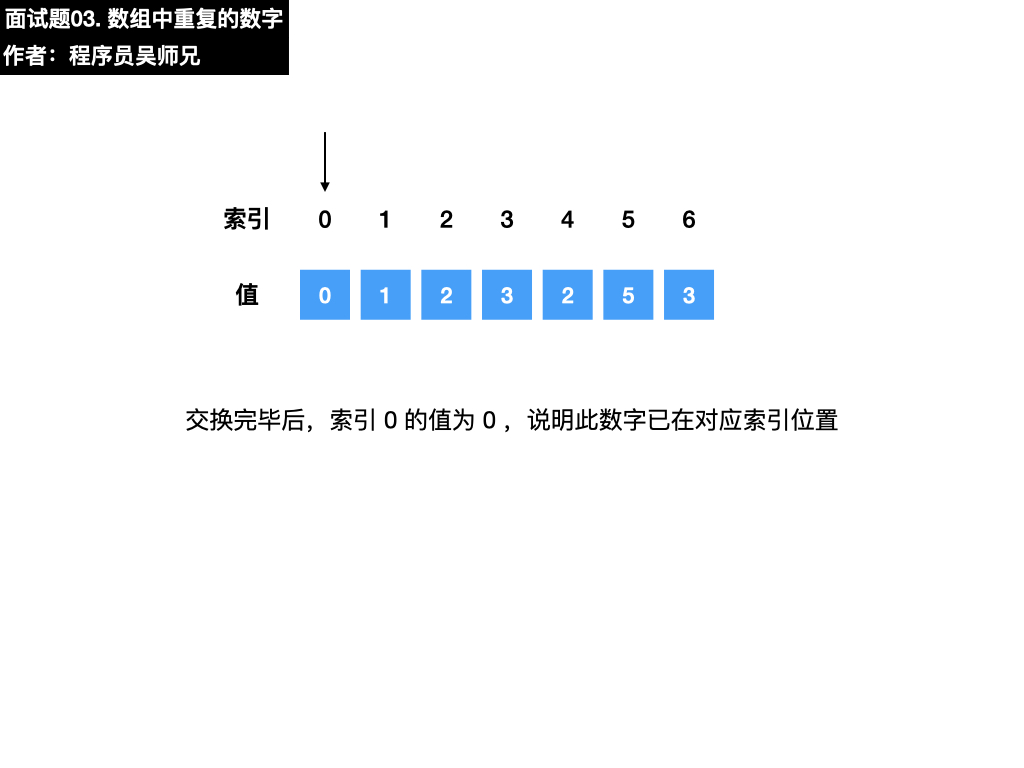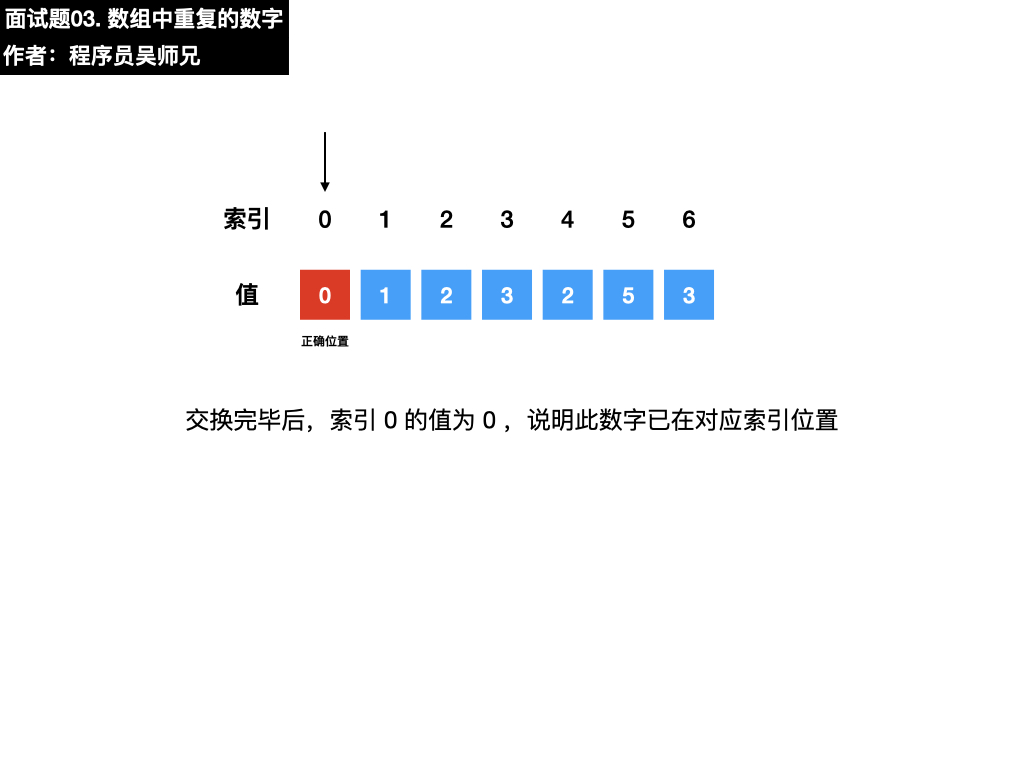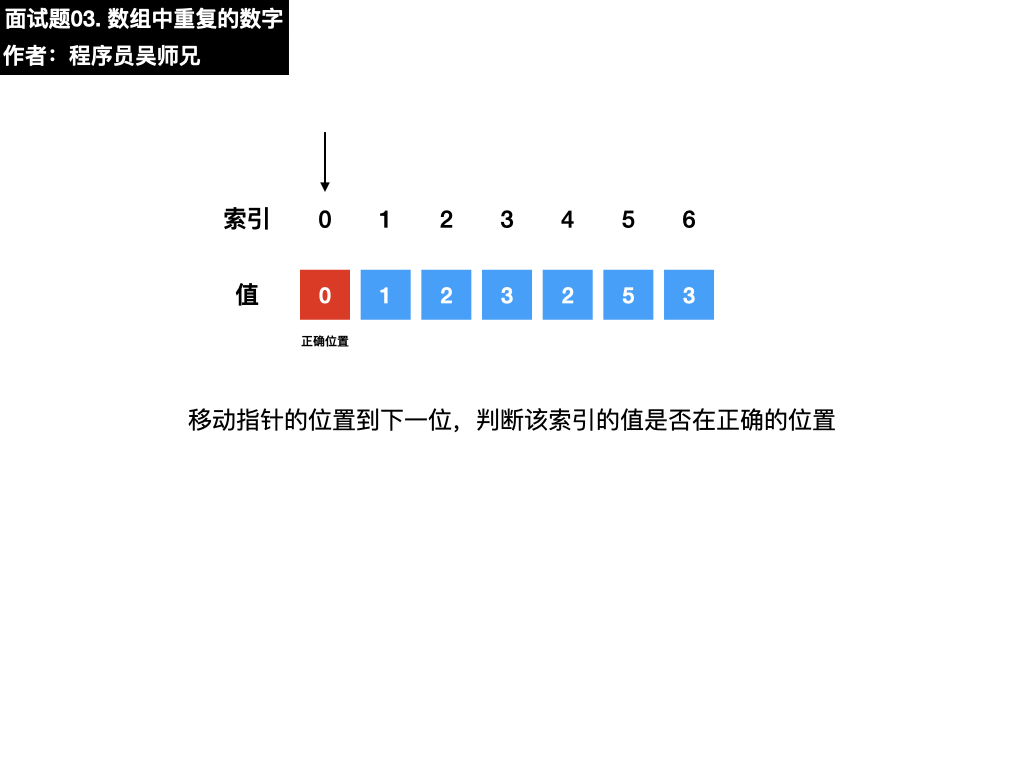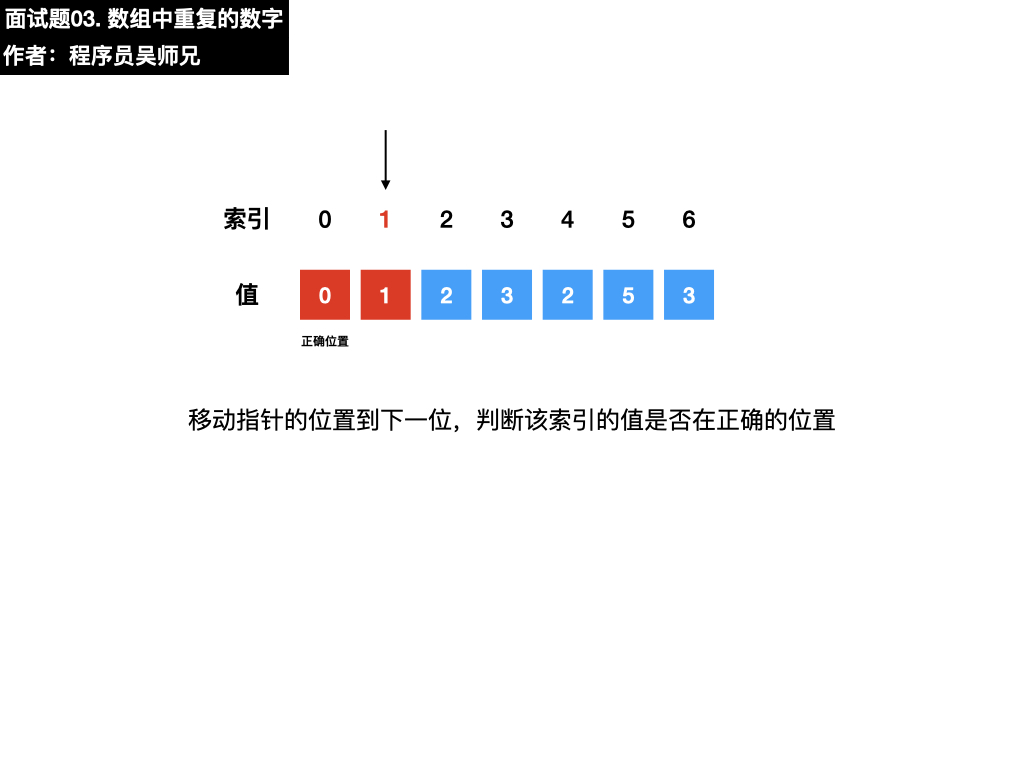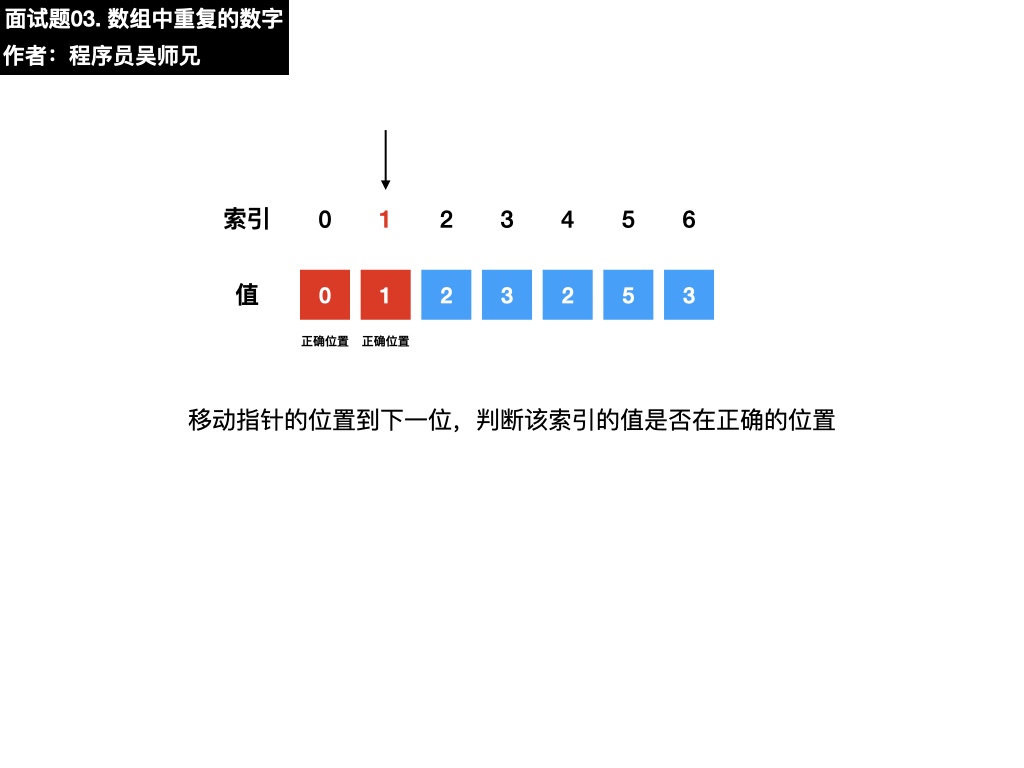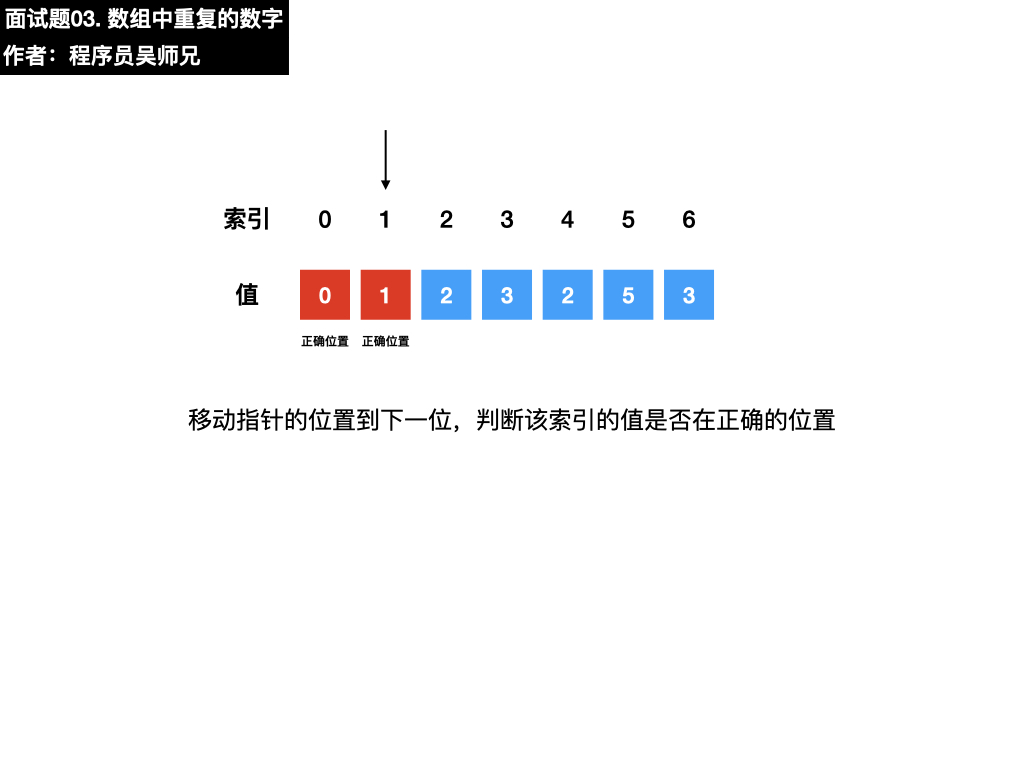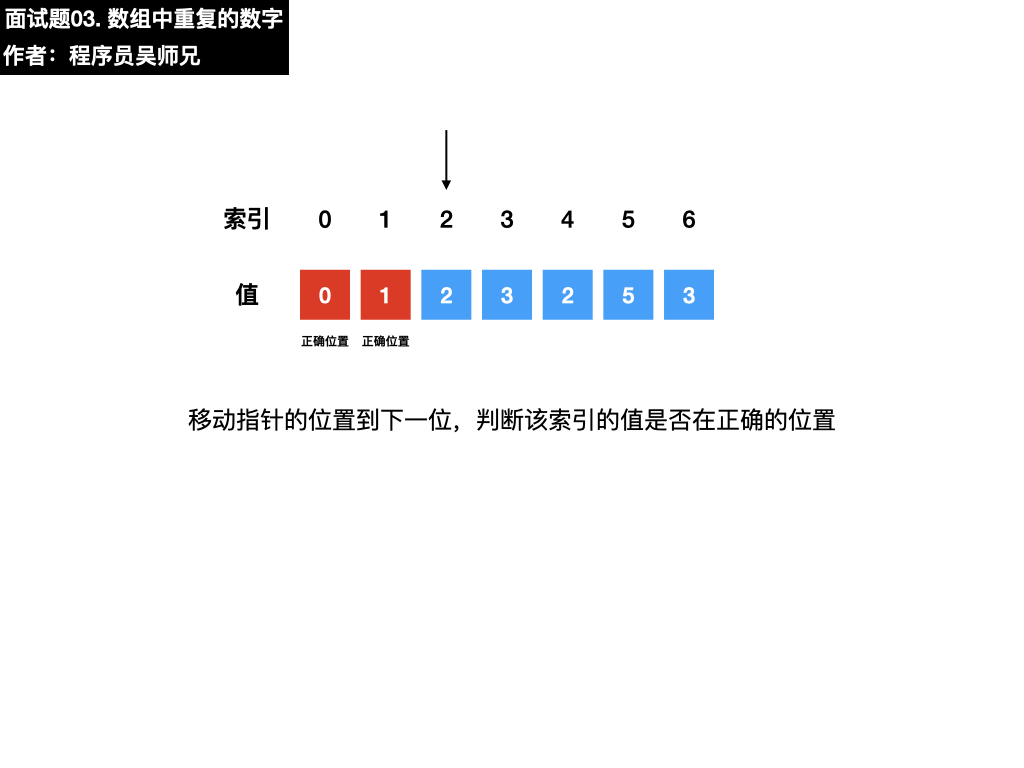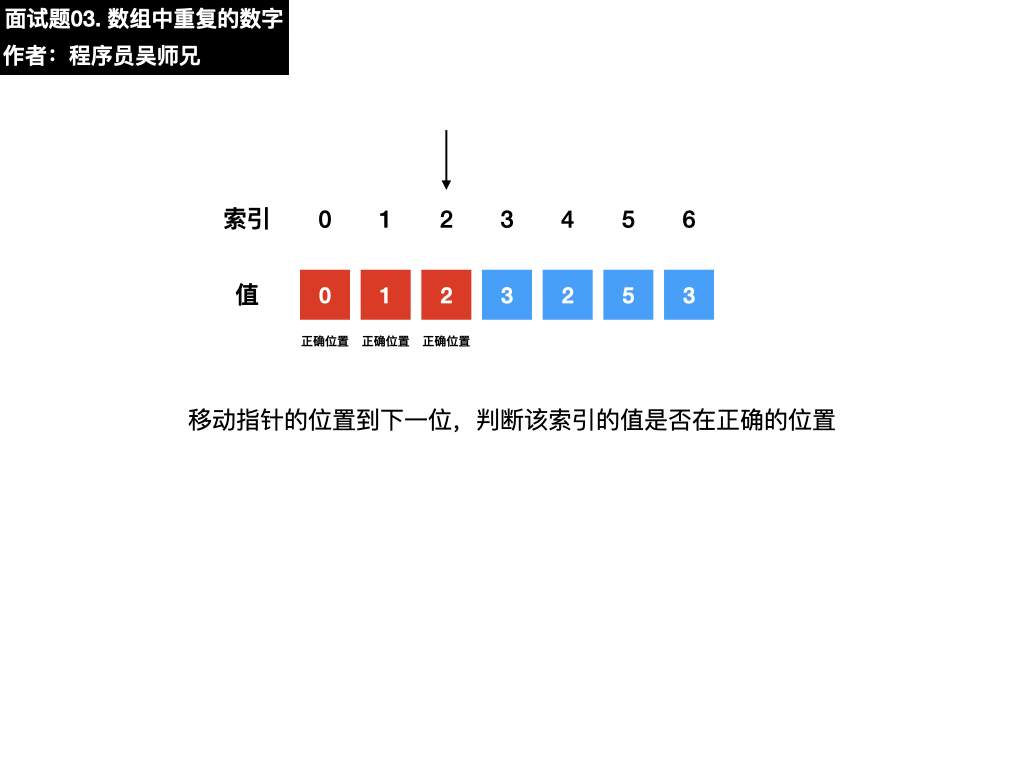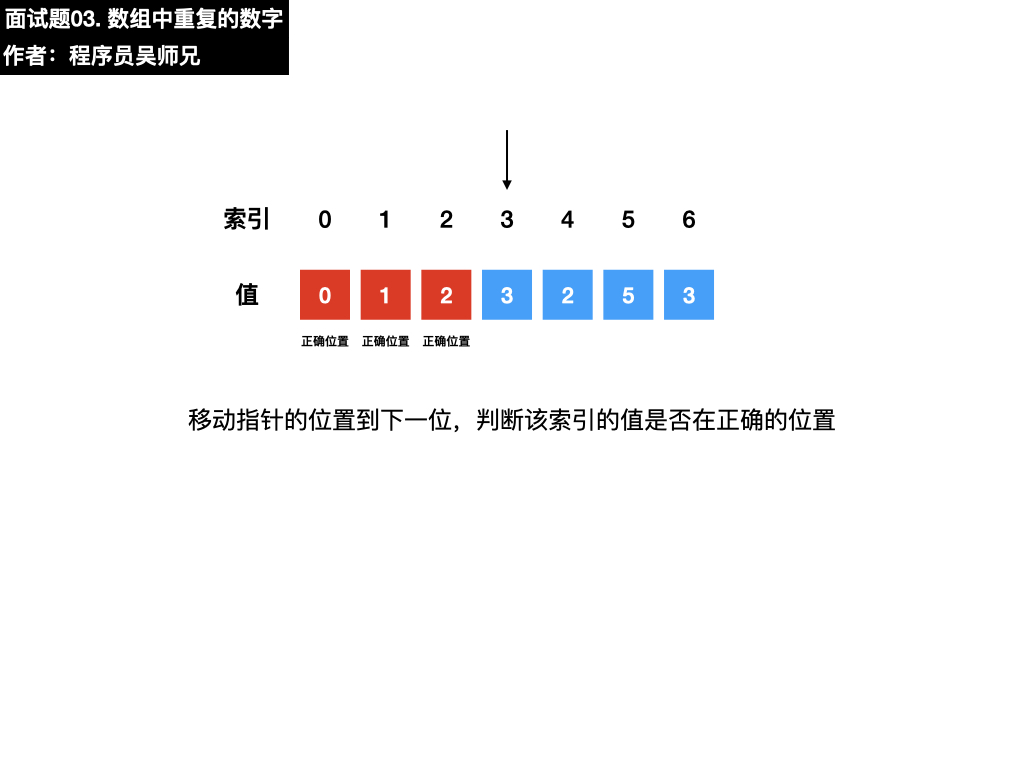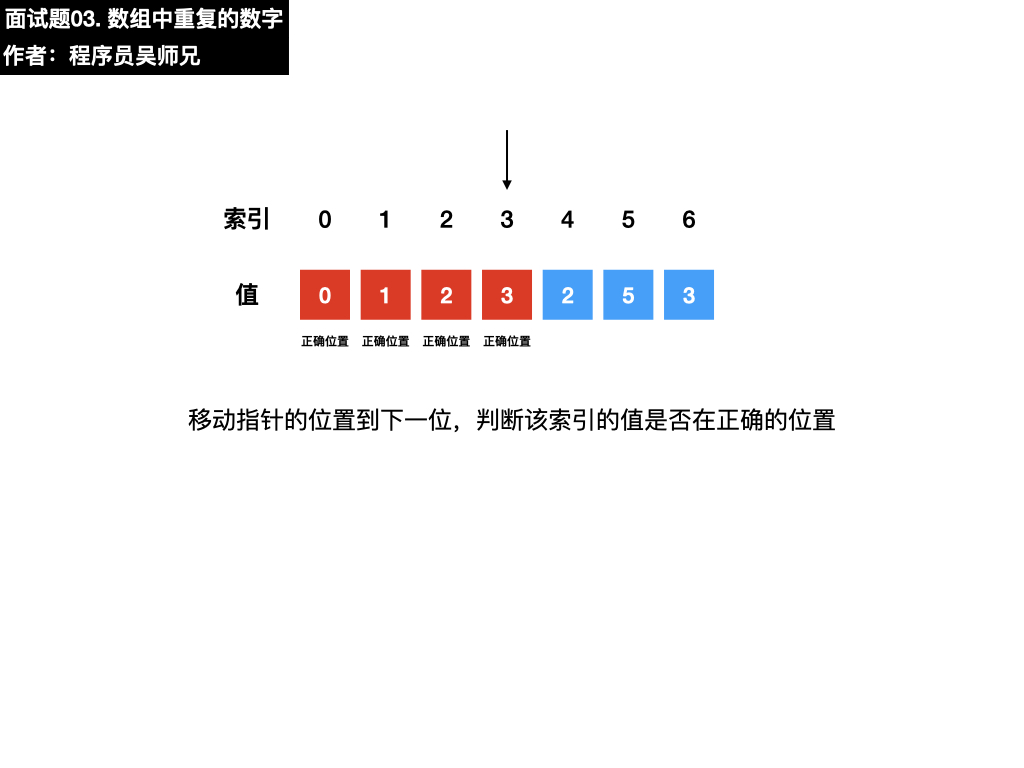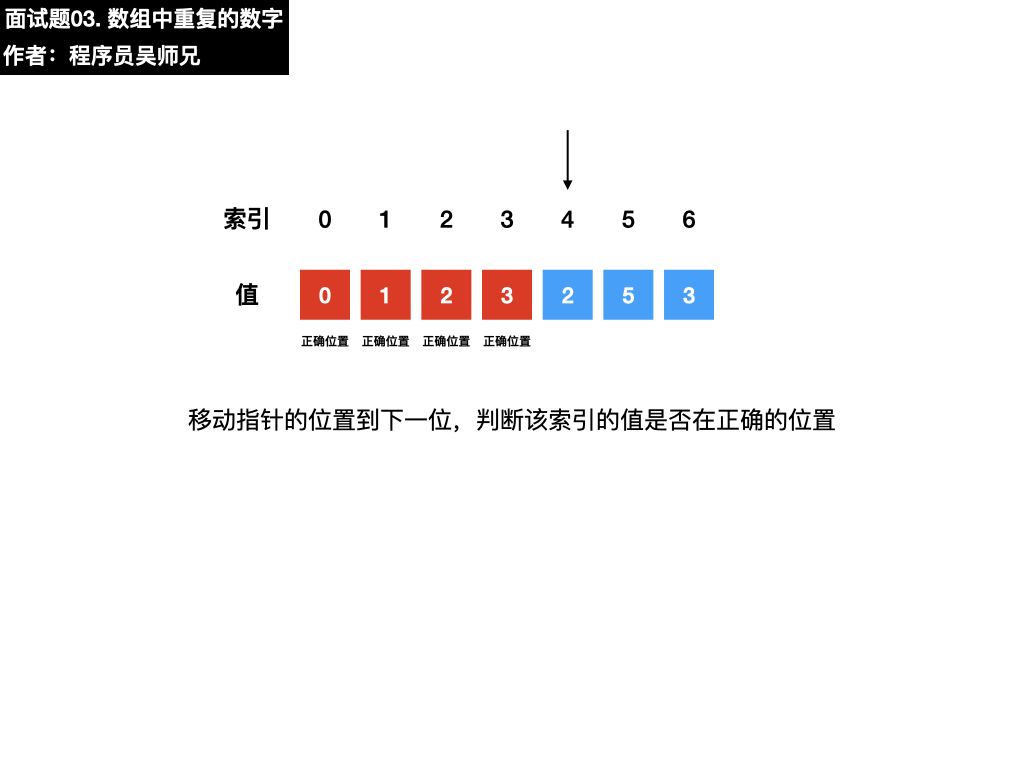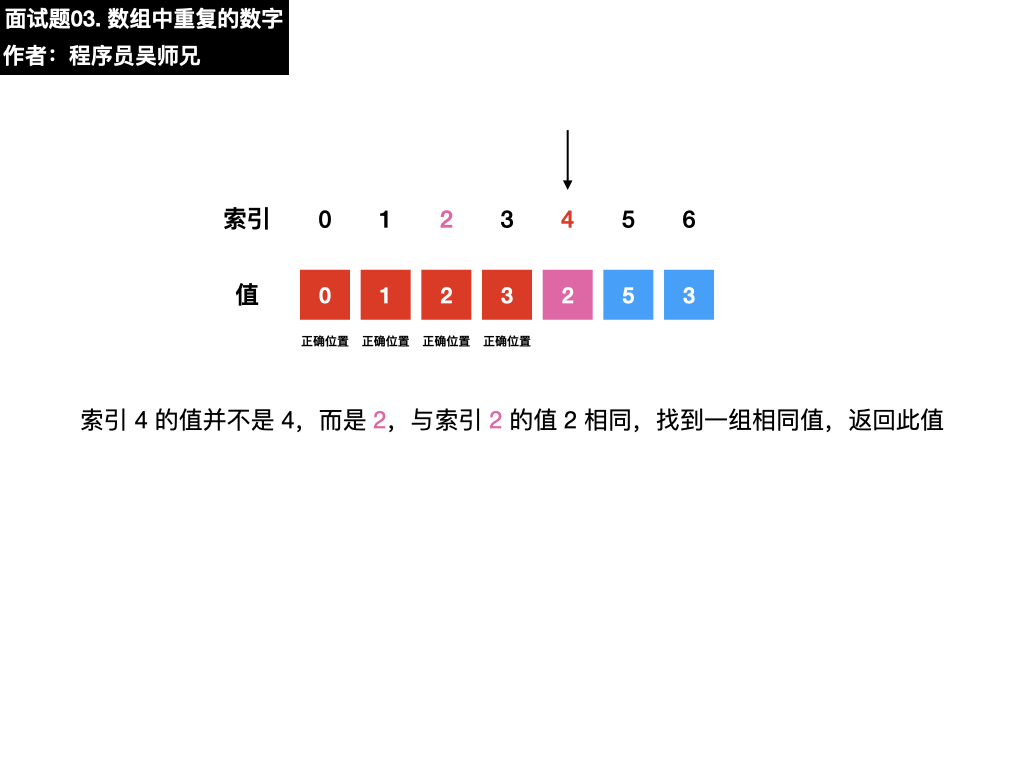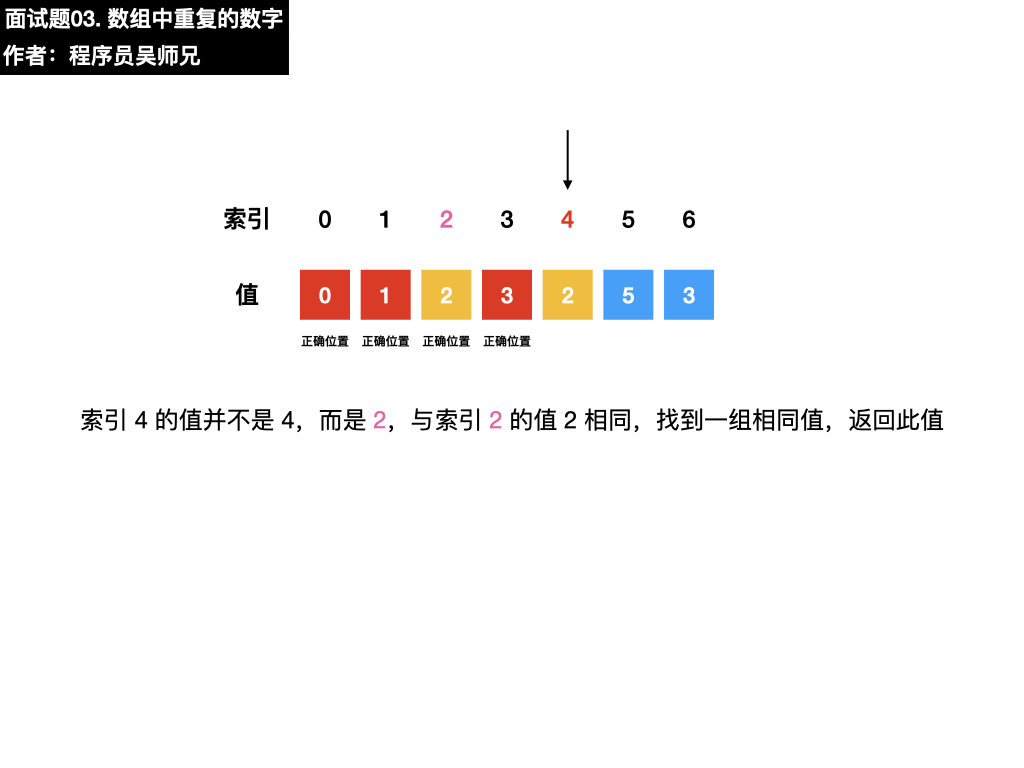### 五、参考代码

class Solution {
public int findRepeatNumber(int[] nums) {
//设索引初始值为 i = 0
int i = 0;
//遍历整个数组 nums
while(i < nums.length) {
//索引 i 的值为 i,无需执行交换操作，查看下一位
if(nums[i] == i) {
i++;
continue;
}
//索引 nums[i] 处的值也为 nums[i]，即找到一组相同值，返回 nums[i] 即可
if(nums[nums[i]] == nums[i]) return nums[i];
//执行交换操作，目的是为了使索引与值一一对应，即索引 0 的值为 0，索引 1 的值为 1
int tmp = nums[i];
nums[i] = nums[tmp];
nums[tmp] = tmp;
}
//如果遍历整个数组都没有找到相同的值，返回 -1
return -1;
}
}

• 数组
• 哈希
• 原地哈希